# Population Ecology: Carrying Capacity

Author: Sophia Tutorial
##### Description:

Determine the carrying capacity of a species from a given graph.

(more)### Developing Effective Teams

*No strings attached. This college course is 100% free and is worth 1 semester credit.

46 Sophia partners guarantee credit transfer.

299 Institutions have accepted or given pre-approval for credit transfer.

* The American Council on Education's College Credit Recommendation Service (ACE Credit®) has evaluated and recommended college credit for 33 of Sophia’s online courses. Many different colleges and universities consider ACE CREDIT recommendations in determining the applicability to their course and degree programs.

Tutorial

what's covered
This tutorial will cover the topic of population ecology. We will discuss the formula that models population growth, and explore the two different types of population growth, as well as life tables, another tool used by population ecologists to measure growth.

Our discussion breaks down as follows:

1. Population Ecology
2. Population Growth
3. Exponential Growth Models
4. Logistic Growth Models
5. Life Tables

## 1. Population Ecology

Population is the group of a given species in a specific time and area. Population ecology is the study of those populations, their characteristics, population growth, and what influences that growth. Important characteristics are as follows:

• Abundance: the number of organisms
• Density: the number of individuals in the population per its specified area
• Patterns of Dispersion: the spatial spread of species geographically
• Age Structure: the relative number of different ages within a population
• Sex Ratio: the number of females versus males within a population
• Variability: the differences between organisms within a species

## 2. Population Growth

Population growth is the rate of growth of a species over a period of time. Population growth is usually understood through modeling or the following simple equation:

formula
Population Growth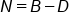In this formula:

• "N" is population change for whatever interval of time is being measured
• "B" is the number of births, also sometimes referred to as fecundity
• "D" is the number of deaths within the population.

Therefore, births minus deaths equals the population change for that time interval.

Positive growth rate occurs when there are more births than deaths. Negative growth occurs when there are more deaths than births, and zero change occurs when the two are equal.

### IN CONTEXT

Let's calculate the fictional population growth of rabbits in Cottonwood, as shown in the graph below.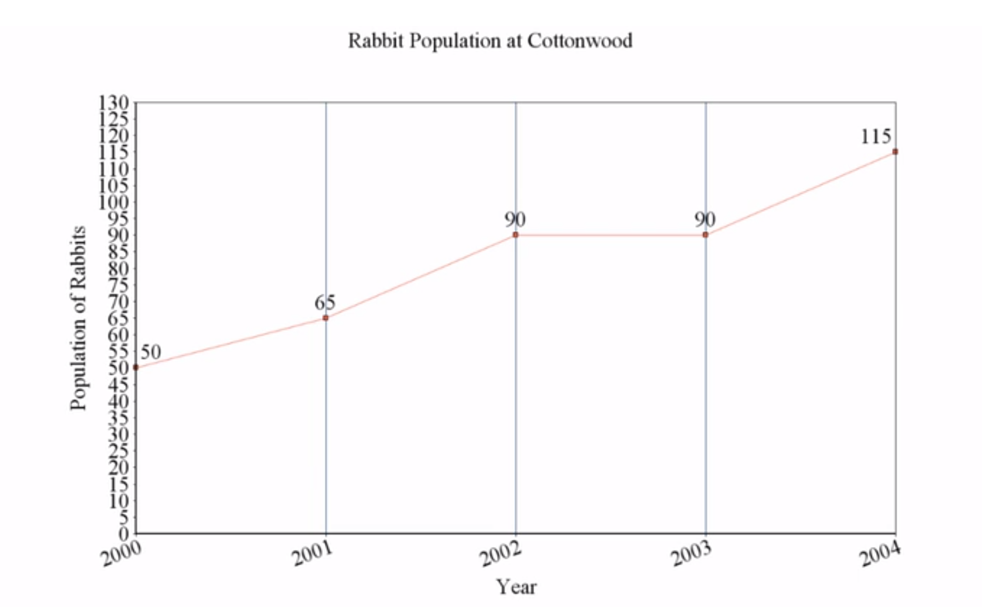The initial population was 50. Suppose in the first year, there were 20 births and five deaths; 20 minus 5 equals 15. Therefore, the population change was plus 15.

You can see that the total population changed from 50 to 65 in 2001, which is an increase of 15.

This process was continued until 2004. The "N", or population change, for 2002 was 25. In 2003, it was zero, and in 2004, it was 25 again.

## 3. Exponential Growth Models

Within the study of population growth, there are two main types: exponential and logistic.

Exponential growth assumes unlimited resources in an idealized situation without limiting factors. Because of this assumption, the population can grow in an exponential manner based on birth and death rates. However, in the real world, exponential growth cannot be sustained for long in any population.

An exponential growth curve when time is the x-axis and population is the y-axis is a lot like a J shape, shown in the diagram below, if extended over enough intervals of time. The slope of an exponential population graph at any given interval is determined by the rate of change of births and deaths.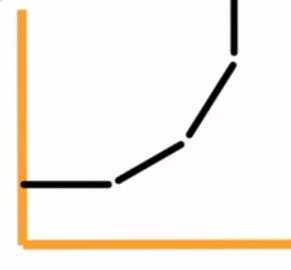EXAMPLE

This first graph is a shallower growth curve, because the population has a lower birth rate. However, if that birth rate increases, the curve of the graph will get steeper.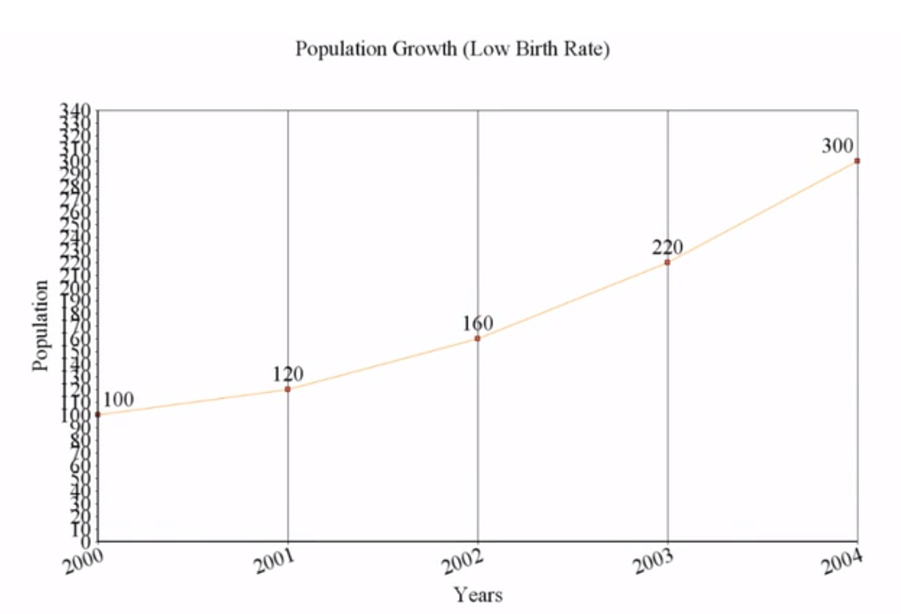EXAMPLE

Next, in the second graph, it is all the same data, except that the birth rate is higher. Can you see how the curve of the graph has gotten steeper? Even though infinite exponential growth is unrealistic, it gives scientists the ability to understand maximum potential population growth in ideal conditions of a species.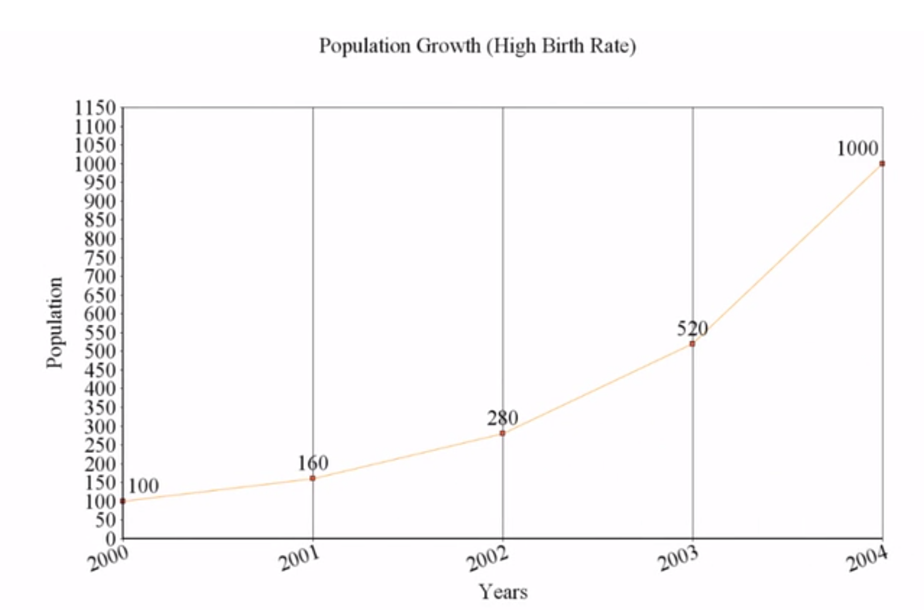## 4. Logistic Growth Models

On the other, more realistic side of things is a logistic population growth graph, which takes into account limited available resources. Limiting resources might be both biotic and abiotic factors, such as food availability or the amount of precipitation.

Due to limiting factors, all populations in a certain area have what is called a carrying capacity, also referred to as "K". This is the maximum sustainable population that an area can support. If a population exceeds this benchmark, it will inevitably decrease and fall below this line, because the ecosystem cannot support anything above that line.

EXAMPLE

Consider the graphical example below. Note the dotted line representing the carrying capacity, or "K". Logistic graphs tend to create an S curve as the population's growth rate increases, and then decreases, as it nears K. In ecosystems, species populations are generally below the carrying capacity for that species in that location.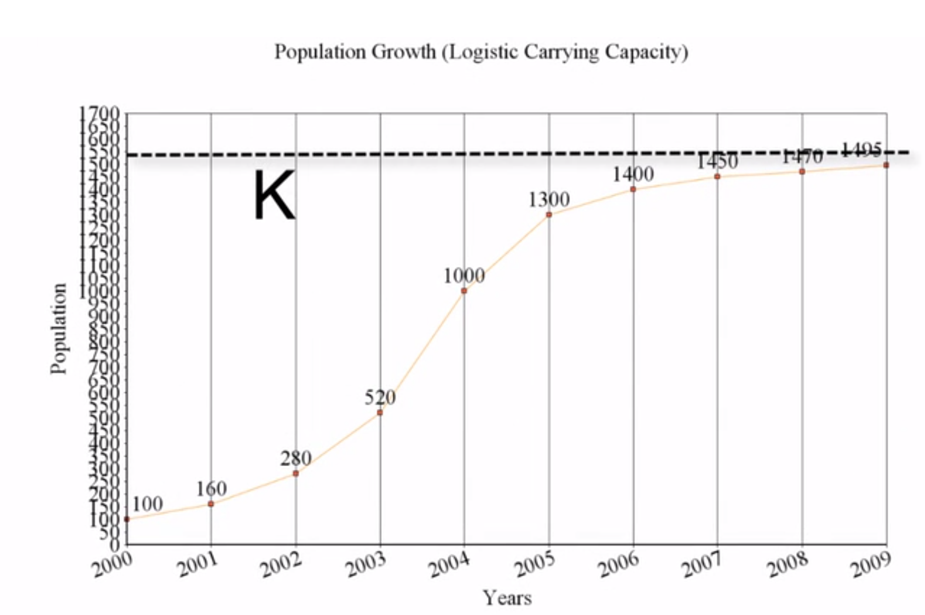## 5. Life Tables

Another important tool for population ecologists is a life table. Life tables, like the one below, summarize age-specific birth and death rates for a species population.

Life Chart of a Fictional Insect
Age (Months) Number Alive Fecundity
0 843 0
1 722 300
2 527 620
3 316 430
4 144 210
5 54 60
6 15 30
7 3 10
8 0

This table shows the number of fictional insects of a particular species at various ages. At one month, it has dropped down to 722 individuals. Its birth rate at three months is 430 offspring.

Life tables like these are used for population management and conservation. It allows humans to identify which parts of species populations at which times are needing protection and which can be harvested.

summary
Today we learned about population ecology and population growth, including the formula that models population growth. We discussed the two types of population growth graphs: exponential and logistic. We also learned about life tables, another important tool for population ecologists, that summarize age-specific birth and death rates for a species population.

Source: Adapted from Sophia instructor Jensen Morgan, GRAPHS CREATED BY JENSEN MORGAN

Rating